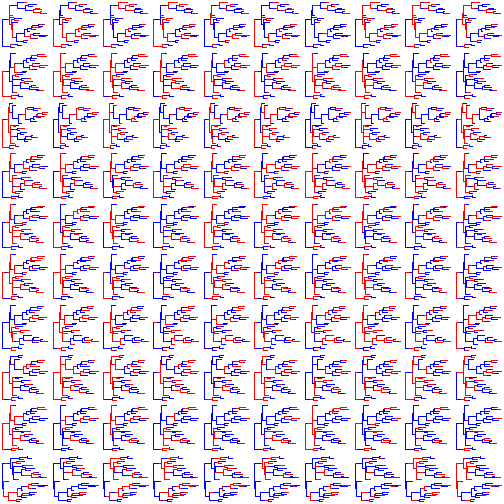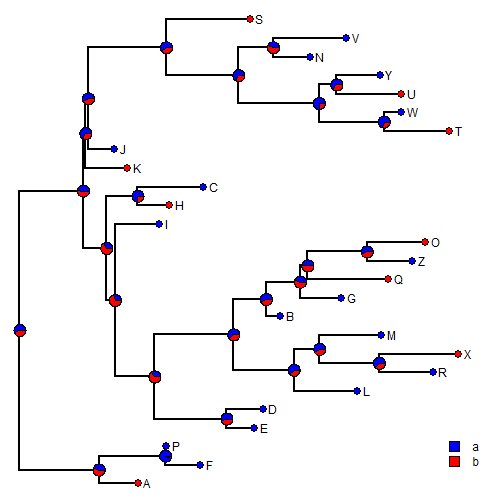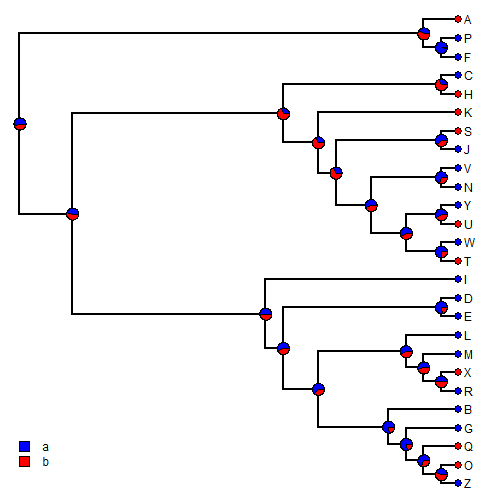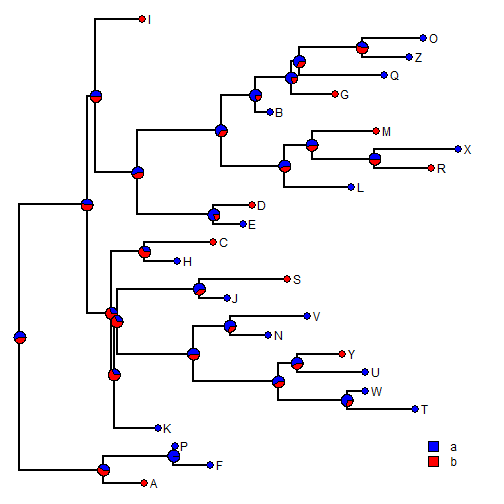## Wednesday, November 4, 2015

### describe.simmap when summarizing the results from stochastic map trees with incongruent topologies

A recent R-sig-phyo user reported a problem with `describe.simmap` in phytools when trying to use it to summary the results from stochastic mapping across multiple trees with potentially incongruent topologies. This is unsurprising to me because the method is not intended for use with such a set of trees - and it assumes, in fact, that all trees are identical. (It does not check for this by default since this is quite slow - but this is an option is `check.equal` is set to `TRUE`.)

For a while, however, I have been meaning to update the function to be able to handle trees with incongruent topologies - by, for example, matching nodes across trees & then only computing the frequency of being in each state for nodes present in the consensus topology or a reference tree.

I just posted exactly this update; however users are cautioned that this has not yet been thoroughly tested. Here is a quick demo:

``````library(devtools)
install_github("liamrevell/phytools")
``````
``````## load packages
library(phytools)
## here are our trees:
trees
``````
``````## 100 phylogenetic trees with mapped discrete characters
``````
``````## they are incongruent:
par(mfrow=c(10,10))
colors<-setNames(c("blue","red"),c("a","b"))
plot(trees,lwd=1,ftype="off",colors=colors)
``````OK, now let's use the method. First, without checking for equality:

``````obj<-summary(trees)
obj
``````
``````## 100 trees with a mapped discrete character with states:
##  a, b
##
## trees have 41.23 changes between states on average
##
## changes are of the following types:
##        a,b   b,a
## x->y 19.51 21.72
##
## mean total time spent in each state is:
##               a          b    total
## raw  15.1882814 11.8398964 27.02818
## prop  0.5619425  0.4380575  1.00000
``````
``````plot(obj,colors=colors)
``````It may not be completely obvious - but close examination reveals that this is not a good result. For instance - tip states do not even necessarily match the input values:

``````x
``````
``````##   A   F   P   K   T   W   U   Y   N   V   J   S   H   C   E   D   L   R
## "b" "a" "a" "b" "b" "a" "b" "a" "a" "a" "a" "b" "b" "a" "a" "a" "a" "a"
##   X   M   B   G   Q   Z   O   I
## "b" "a" "a" "a" "b" "a" "b" "a"
``````

OK, now let's check if the trees are equal. In the new phytools version of this function when trees are not equal, a consensus tree is built which is then used to compute posterior probabilities for nodes (that occur in the consensus tree). Of course, we will lose our branch lengths:

``````obj<-summary(trees,check.equal=TRUE) ## much slower
``````
``````## Note: Some trees are not equal.
## A "reference" tree will be computed if none was provided.
##
## No reference tree provided & some trees are unequal.
## Computing majority-rule consensus tree.
``````
``````obj
``````
``````## 100 trees with a mapped discrete character with states:
##  a, b
##
## trees have 41.23 changes between states on average
##
## changes are of the following types:
##        a,b   b,a
## x->y 19.51 21.72
##
## mean total time spent in each state is:
##               a          b    total
## raw  15.1882814 11.8398964 27.02818
## prop  0.5619425  0.4380575  1.00000
``````
``````plot(obj,colors=colors)
``````Finally, if we have some kind of pre-existing reference tree, such as (for example) a Bayesian MCC tree, and we want to input it & compute Bayesian posterior probabilities on that tree, we can do it:

``````tree
``````
``````##
## Phylogenetic tree with 26 tips and 25 internal nodes.
##
## Tip labels:
##  A, F, P, K, T, W, ...
##
## Rooted; includes branch lengths.
``````
``````obj<-summary(trees,ref.tree=tree)
obj
``````
``````## 100 trees with a mapped discrete character with states:
##  a, b
##
## trees have 41.23 changes between states on average
##
## changes are of the following types:
##        a,b   b,a
## x->y 19.51 21.72
##
## mean total time spent in each state is:
##               a          b    total
## raw  15.1882814 11.8398964 27.02818
## prop  0.5619425  0.4380575  1.00000
``````
``````plot(obj,colors=colors)
``````That's it!

As warned, I just did this - so there are sure to be bugs. Please report any via email or on R-sig-phylo. Thanks!

1.I found I had to read this in conjunction with http://blog.phytools.org/2013/04/using-makesimmap-on-set-of-trees.html to make sense of it. Also I take that \$ace does not work here?

2.I am having trouble controlling the graphical options after these steps:
obj<-summary(trees,ref.tree=tree)
obj
plot(obj,fsize=0.3,colors=colors,cex=0.1)
This gets the labels in the right size, and the tip symbols but not the pie charts.

I want to modify tip labels, and keep tip symbol small and allow pie charts to be a little bigger. What is plot using for the summary here? How does it interact with object of class "describe.simmap"?

3.Note: due to the very large amount of spam, all comments are now automatically submitted for moderation.